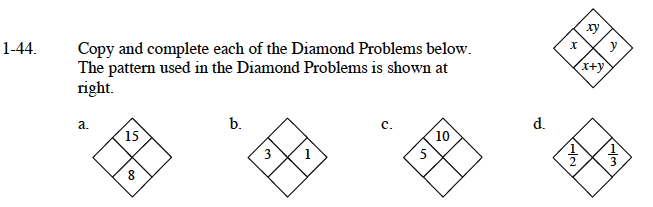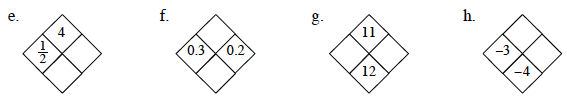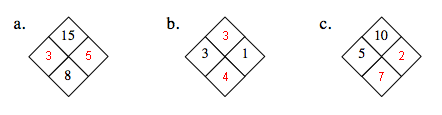### Home > CAAC > Chapter 1 > Lesson 1.2.1 > Problem1-44

1-44.Find out what values are missing from the diamond.(Is the x-value missing? Y-value? XY value? X + y value?)

If the x and y value are missing, find what values can equal the top number, and what values equal the bottom number.

If the xy value and the x + y value are missing, use the values you do have (the x value and the y value) and multiply them together for the top value and add them together for the bottom value.

If the x + y value and the y value are missing: To find y: Find what number multiplied by x equals the top number.To find x + y: Use the y value you found and the x value given and add them together.

If the xy and y value are missing: To find y: Use the x value given and the x+y value and subtract the x value from the x+y value. To find xy: Use the y value you found and the x value given and multiply.